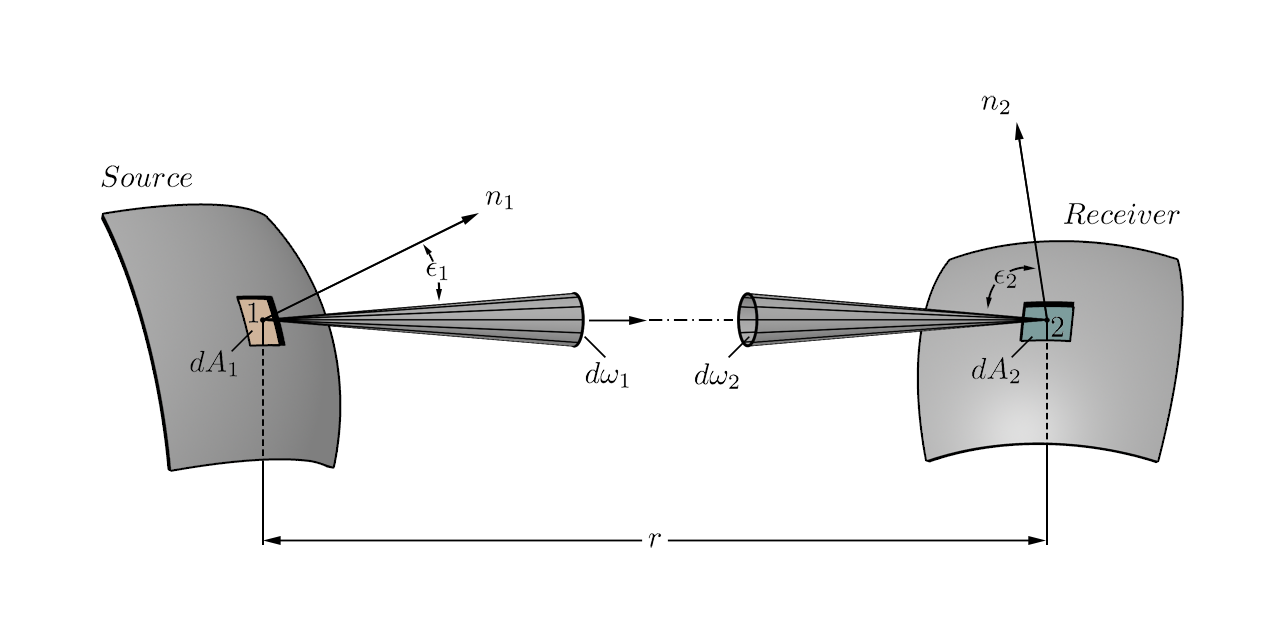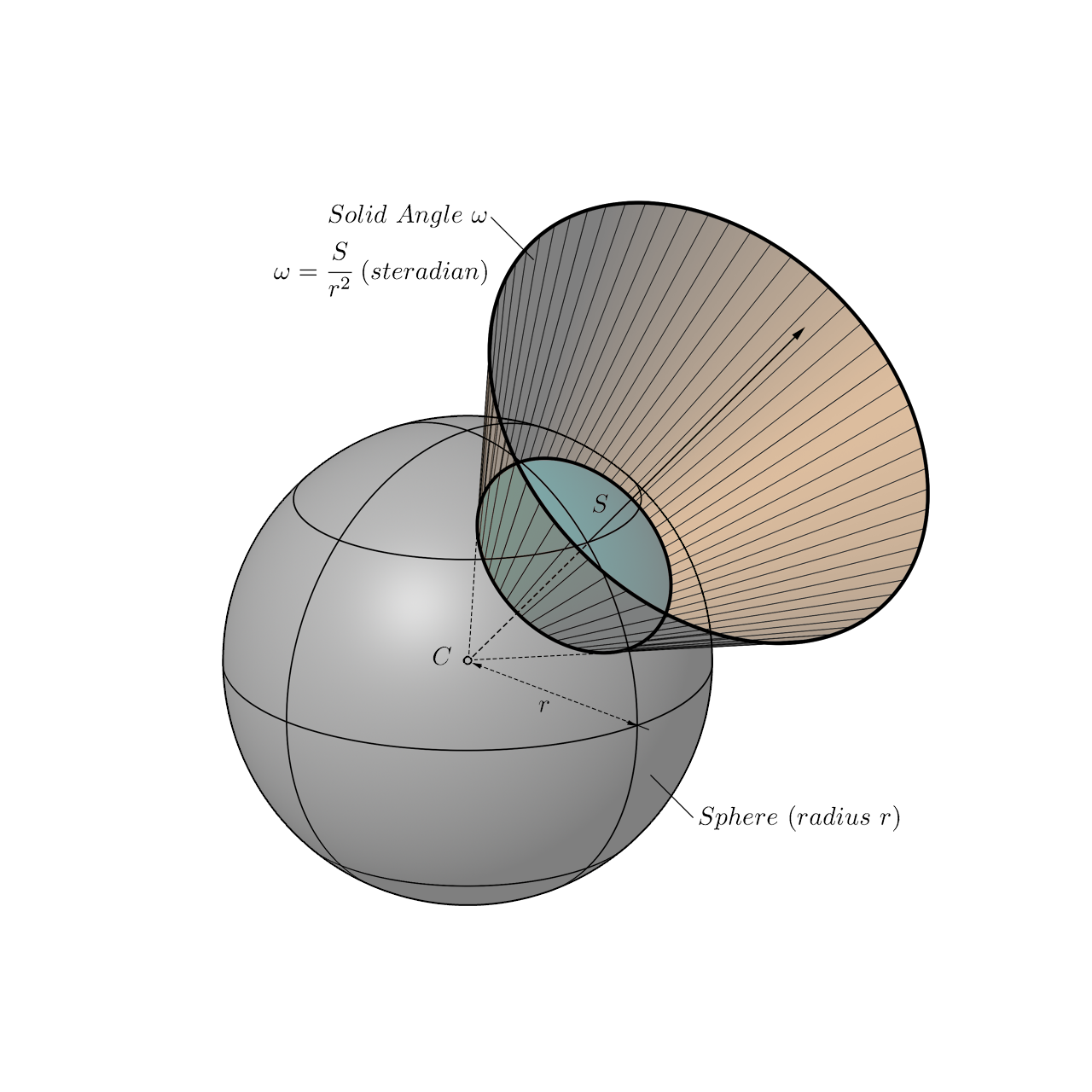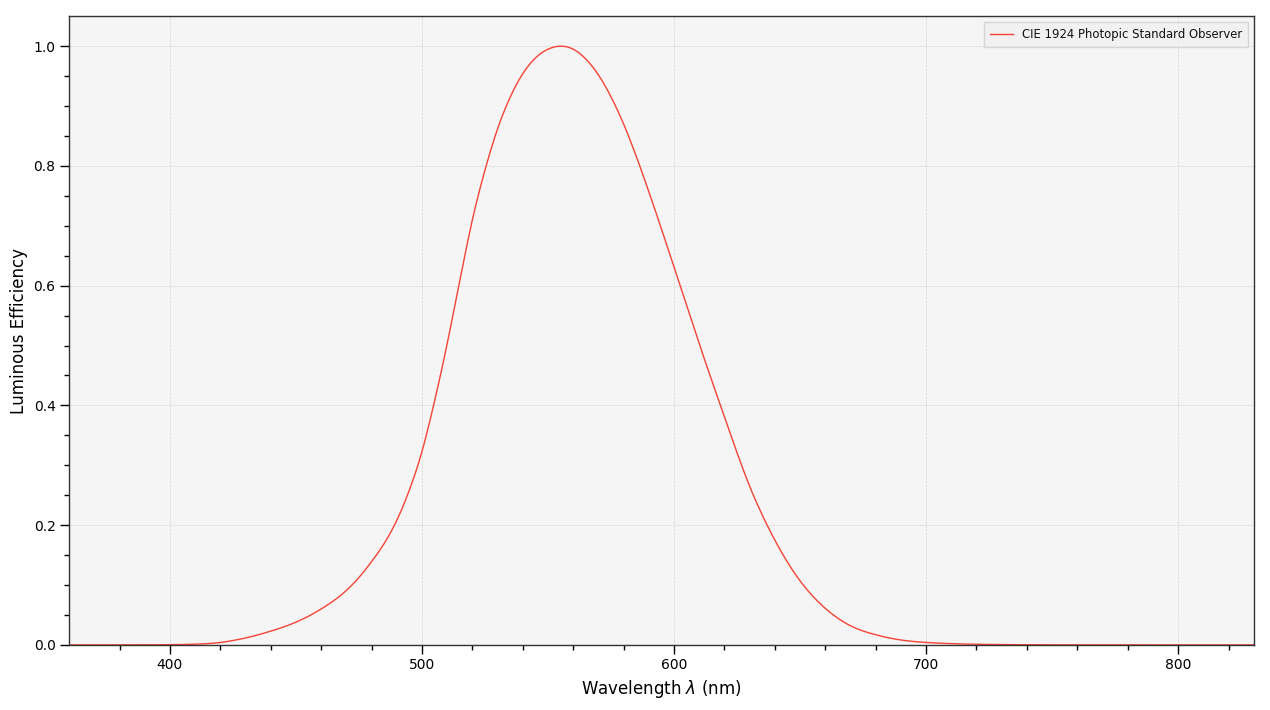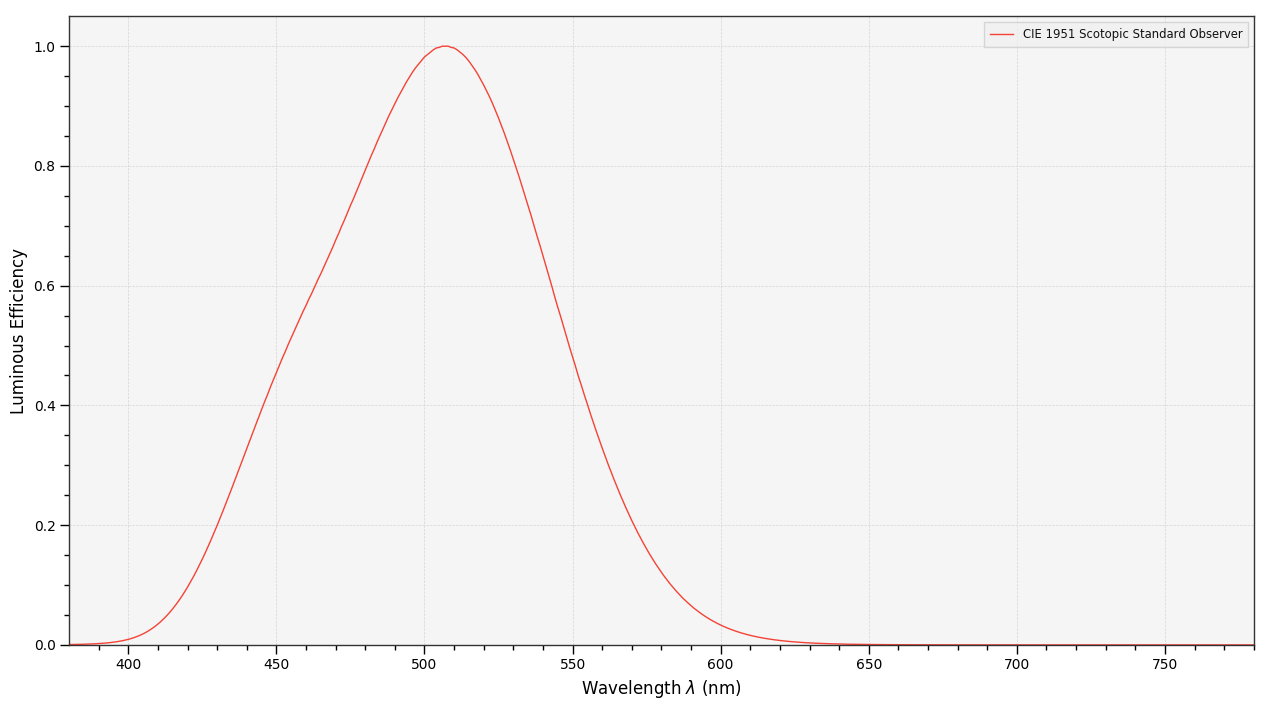Luminous Efficiency Functions¶

The luminous efficiency function is the ratio of radiant flux weighted according to $V(\lambda)$ to the corresponding radiant flux.  It characterizes the average spectral sensitivity of human visual perception of brightness. Brightness is defined as the attribute of a visual perception according to which an area appears to emit, or reflect, more or less light. 

Photometry is the measurement of quantities referring to radiation as evaluated according to a given spectral luminous efficiency function, e.g. $V(\lambda)$ or $V^\prime(\lambda)$. 

Radiometry is defined as the measurement of the quantities associated with optical radiation. 

Photometric quantities are weighted accordingly to human visual system spectral sensitivity within the wavelength range 360-780 nanometres ($nm$) while radiometric quantities represent unweighted absolute power within the wavelength range 0.01-1000 micrometres ($\mu m$).

Given the following terms and units table: 

Term Symbol Defining Equation Units Units Name Notes
Frequency $v$ $s^{-1}$ Hertz
Wavelength $\lambda$ $\lambda=\cfrac{c}{v}$ $m$ Metre $c$: Velocity of radiant energy in vacuum
Wavenumber $m$ $m=\cfrac{1}{\lambda}$ $m^{-1}$ Metre
Solid angle $\omega$ $\omega=\cfrac{S}{r^2}$ $sr$ Steradian $S$: Portion of sphere surface
$r$: Radius of sphere, also distance between (1) of $dA_1$ and (2) of $dA_2$$\phantom{\ \ \ \ \ \ \ \ \ \ }$$\phantom{\ \ \ \ \ \ \ \ \ \ }$$\phantom{\ \ \ \ \ \ \ \ \ \ }$$\phantom{\ \ \ \ \ \ \ \ \ \ }$$\phantom{\ \ \ \ \ \ \ \ \ \ }$$\phantom{\ \ \ }$

Radiometry uses the following quantities, terms and units: 

Quantity / Term Symbol Defining Equation Units Units Name Notes
Radiant energy $Q_e$ $J$ Joule
Radiant flux (or power) $P_e$ $W$ Watt
($J\cdot sr^{-1}$)
$d^2P_e=L_e\cfrac{dA_1\cos\varepsilon_1dA_2\cos\varepsilon_2}{r^2}$
Radiant exitance $M_e$ $M_e=\cfrac{dP_e}{dA_1}$ $W\cdot m^{-2}$ Watt per square metre $dA_1$: Surface element of source
Irradiance $E_e$ $E_e=\cfrac{dP_e}{dA_2}$ $W\cdot m^{-2}$ Watt per square metre $dA_1$: Surface element of receiver
Radiant intensity $I_e$ $I_e=\cfrac{dP_e}{d\omega_1}$ $W\cdot sr^{-1}$ Watt per steradian $d\omega_1$: Element of solid angle with apex (2) at surface of source
Radiance $L_e$ $L_e=\cfrac{d^2P_e}{dA_1\cos\varepsilon_1d\omega_1}$
$L_e=\cfrac{d^2E_e}{dA_2\cos\varepsilon_2d\omega_2}$
$L_e=\cfrac{d(E_e)_n}{d\omega_2}$
$W\cdot m^{-2} \cdot sr^{-1}$ Watt per square metre per steradian $\varepsilon_1$: Angle between direction (1)-(2) and normal $n_1$ of $dA_1$
$\varepsilon_2$: Angle between direction (1)-(2) and normal $n_2$ of $dA_2$
$d\omega_2$: Element of solid angle with apex (2) at surface of receiver
$dA_1\cos\varepsilon_1$: $dA_1$ orthogonally projected on plane perpendicular to direction (1)-(2)
$dA_2\cos\varepsilon_2$: $dA_2$ orthogonally projected on plane perpendicular to direction (1)-(2)
$d(E_e)_n=\cfrac{dE_e}{dA_2\cos\varepsilon_2}$
$d\omega_1=\cfrac{dA_1\cos\varepsilon_1}{r^2}$
$d\omega_2=\cfrac{dA_2\cos\varepsilon_2}{r^2}$

Photometry uses the following quantities, terms and units: 

Quantity / Term Symbol Defining Equation Units Units Name Notes
Luminous energy $Q_v$ $lm\cdot s$ Lumen second
Luminous flux (or power) $F_v$ (or $P_v$) $F_v=KP_e$
$F_v=K_m\int_\lambda P_{e,\lambda}V(\lambda)d\lambda$
$lm$ Lumen
($cd\cdot sr$)
$P_e$: Radiant flux ($W$)
$K$: Luminous efficacy ($lm\cdot W^{-1}$)
Luminous exitance $Mv$ $M=\cfrac{dF_v}{dA_1}$ $lx$ Lumen per square metre (or lux)
($lm\cdot m^{-2}$)
Illuminance $E_v$ $E_v=\cfrac{dF_v}{dA_2}$ $lx$ Lumen per square metre (or lux)
($lm\cdot m^{-2}$)
$dA_2$: Surface element of receiver
Luminous intensity $I_v$ $I_v=\cfrac{dF_v}{d\omega_I}$ $cd$ Candela
($lm\cdot sr^{-1}$)
Luminous flux per unit solid angle
$d\omega_I$: Element of solid angle with apex (1) at surface of source
Luminance $L_v$ $L_v=\cfrac{d^2F_v}{dA_1\cos\varepsilon_1d\omega_1}$
$L_v=\cfrac{dI_v}{dA_1\cos\varepsilon_1}$
$L_v=\cfrac{d^2E_v}{dA_2\cos\varepsilon_2d\omega_2}$
$L_v=\cfrac{dE_{v,n}}{d\omega_2}$
$cd\cdot m^{-2}$ Candela per square metre (or nits)
($lm\cdot m^{-2}\cdot sr^{-1}$)
$dA_1$: Surface element of source
$\varepsilon_1$ Angle between direction (1)-(2) and normal $n_1$ of $dA_1$
$dA_1\cos\varepsilon_1$: $dA_1$ orthogonally projected on plane perpendicular to direction (1)-(2)
$dE_{v, n}$: Illuminance on $dA_2$ normal to the direction (1)-(2)
$d\omega_2$: Element of solid angle with apex (2) at surface of receiver
Luminous efficacy function $K(\lambda)$ $K(\lambda)=K_mV(\lambda)$
with $K_m$=683
$V(\lambda)$: Relative photopic luminous efficiency function

The following figures illustrate the notes from the above tables: 

In :
from IPython.core.display import Image

Image(filename='resources/images/Photometric_Quantities_001.png')
Out:In :
from IPython.core.display import Image

Image(filename='resources/images/Solid_Angle_001.png')
Out:As per the above tables, a quantity in the photometric system has a similar quantity in the radiometric system:

• Radiant energy and luminous energy.
• Radiant flux and luminous flux.
• Radiance exitance and luminous exitance.
• Radiant intensity and luminous intensity.

Photoreceptors¶

The human eye has two different types of photoreceptor cells:

• The cone cells are mediating the photopic vision, responsible for the colour perception and defined as the vision by the normal eye in which cones are the principle active photoreceptors.  In the photoreceptor layer of the retina, around $6.8\cdot 10^6$ cone cells of types $S$, $M$, $L$ are measuring light with respective peak absorption at wavelengths of about 420 nm, 534 nm and 564 nm. Photopic vision luminance levels are defined for $L_v>10\ cd\cdot m^{-2}$.
• The rod cells are mediating the scotopic vision, the vision under dim light conditions and defined as the vision by the normal eye in which rods are the principle active photoreceptors.  There is an average of $110-125\cdot 10^6$ rod cells in the retina although none are located in the central fovea region. Scotopic vision luminance levels are defined for $L_v<0.01\ cd\cdot m^{-2}$.

Luminous Efficiency Functions¶

Colour provides the following photopic and scotopic luminous efficiency functions:

In :
import colour

sorted(colour.LEFS.keys())
Out:
['CIE 1924 Photopic Standard Observer',
'CIE 1951 Scotopic Standard Observer',
'CIE 1964 Photopic 10 Degree Standard Observer',
'CIE 2008 10 Degree Physiologically Relevant LEF',
'CIE 2008 2 Degree Physiologically Relevant LEF',
'Judd Modified CIE 1951 Photopic Standard Observer',
'Judd-Vos Modified CIE 1978 Photopic Standard Observer',
'cie_10_1964',
'cie_1951',
'cie_2_1924']

Note: 'cie_2_1924', 'cie_10_1964' and 'cie_1951' are convenient aliases for respectively 'CIE 1924 Photopic Standard Observer', 'CIE 1964 Photopic 10 Degree Standard Observer' and 'CIE 1951 Scotopic Standard Observer'.

CIE 1924 Photopic Standard Observer¶

The CIE 1924 Photopic Standard Observer luminous efficiency function $V(\lambda)$ was established by the CIE in 1924 for a 2° angular subtense viewing field. It was derived from several independent experiments whose results were not averaged but weight assembled by Gibson and Tyndall (1923) from very different sets of data: 

• Minimum flicker experiments by Coblentz and Emerson (1918)
• Step-by-step experiments by Hyde, Forsythe and Cady (1918) and Gibson and Tyndall (1923)
• Direct brightness matching experiments by Hyde, Forsythe and Cady (1918) and Hartman (1918)

The angular subtense viewing field size varied among those experiments and the surround ﬁeld was not always of constant luminance, if present at all. The assembly of data gathered from very different experiments has subsequently affected the effectiveness of the luminous efficiency function $V(\lambda)$. 

Photopic luminous flux $P_v$ and radiant flux $P_e$ are related by the following equation: 

$$P_v=K_m\int_\lambda P_{e,\lambda}V(\lambda)d\lambda$$

Accordingly, the photopic luminous efficacy $K$ defined as follows:

$$K(\lambda)=\cfrac{P_{v,\lambda}}{P_{e,\lambda}}$$

is equal to:

$$K(\lambda)=K_mV(\lambda)$$

with $K_m=683\ lm\cdot W^-1$ at $\lambda_d=555\ nm$.

The CIE 1924 Photopic Standard Observer luminous efficiency function $V(\lambda)$ has the following range and increments:

In :
colour.PHOTOPIC_LEFS['CIE 1924 Photopic Standard Observer'].shape
Out:
SpectralShape(360.0, 830.0, 1.0)
In :
from colour.plotting import *
In :
colour_style();
In :
plot_multi_sds(
[colour.PHOTOPIC_LEFS['CIE 1924 Photopic Standard Observer']],
y_label='Luminous Efficiency');CIE 1951 Scotopic Standard Observer¶

The CIE 1951 Scotopic Standard Observer luminous efficiency function $V^\prime(\lambda)$ was established by the CIE in 1951 and is a relative function of wavelength with peak at $\lambda_d=507\ nm$.

Scotopic luminous flux $P_v^\prime$ and radiant flux $P_e$ are related by the following equation: 

$$\begin{equation} P_v^\prime=K_m\int_\lambda P_{e,\lambda}^\prime V(\lambda)d\lambda \end{equation}$$

Accordingly, the scotopic luminous efficacy $K^\prime$ defined as follows:

$$\begin{equation} K^\prime(\lambda)=\cfrac{P_{v,\lambda}^\prime}{P_{e,\lambda}} \end{equation}$$

is equal to:

$$\begin{equation} K^\prime(\lambda)=K_m^\prime V(\lambda) \end{equation}$$

with $K_m=1700\ lm\cdot W^-1$ at $\lambda_d=507\ nm$.

The CIE 1951 Scotopic Standard Observer luminous efficiency function $V^\prime(\lambda)$ has the following range and increments:

In :
colour.SCOTOPIC_LEFS['CIE 1951 Scotopic Standard Observer'].shape
Out:
SpectralShape(380.0, 780.0, 1.0)
In :
plot_multi_sds(
[colour.SCOTOPIC_LEFS['CIE 1951 Scotopic Standard Observer']],
y_label='Luminous Efficiency');Mesopic Vision¶

Mesopic vision is a combination of photopic vision and scotopic vision and is defined for for $L_v\pm 0.2\ cd\cdot m^{-2}$. Although there is no standard for mesopic vision, a weighting function $V_m(\lambda)$ is defined as follows: 

$$\begin{equation} V_m(\lambda)=(1-x)V^\prime(\lambda)+xV(\lambda) \end{equation}$$

where $x$ is function of photopic luminance $L_p$ and can have value of:

$L_p$ Blue-heavy
(MOVE)
Blue-heavy
(LRC)
Red-heavy
(MOVE)
Red-heavy
(LRC)
0.01 0.13 0.04 0.00 0.01
0.1 0.42 0.28 0.34 0.11
1.0 0.70 1.00 0.68 1.00
10 0.98 1.00 0.98 1.00

The MOVE is an european research consortium and LRC stands for Lighting Research Center.

The colour.mesopic_weighting_function implements support for mesopic vision following the above equation:

In :
colour.colorimetry.mesopic_weighting_function(wavelength=500, Lp=0.2)
Out:
0.70522000000000018

We can use different parameters for $x$:

In :
colour.colorimetry.mesopic_weighting_function(500, 0.2, source='Red Heavy', method='LRC')
Out:
0.90951000000000015

Conveniently the colour.sd_mesopic_luminous_efficiency_function definition implements the creation of a mesopic luminous efficiency function based on colour.mesopic_weighting_function definition:

In :
# Plotting a mesopic luminous efficiency function with photopic luminance of 0.2.
sd_mesopic_luminous_efficiency_function = colour.sd_mesopic_luminous_efficiency_function(0.2)

plot_multi_sds(
[sd_mesopic_luminous_efficiency_function,
colour.PHOTOPIC_LEFS['CIE 1924 Photopic Standard Observer'],
colour.SCOTOPIC_LEFS['CIE 1951 Scotopic Standard Observer']],
y_label='Luminous Efficiency');